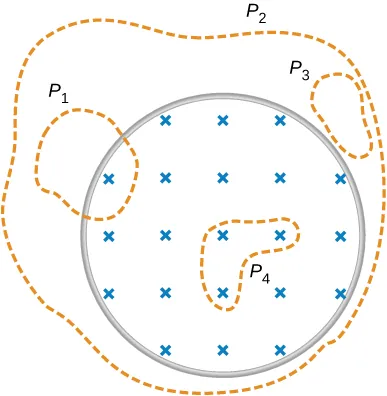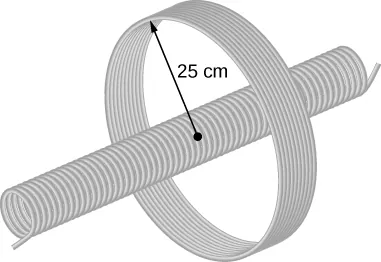University Physics Volume 2

# 13.4Induced Electric Fields

University Physics Volume 213.4 Induced Electric Fields

### Learning Objectives

By the end of this section, you will be able to:

• Connect the relationship between an induced emf from Faraday’s law to an electric field, thereby showing that a changing magnetic flux creates an electric field
• Solve for the electric field based on a changing magnetic flux in time

The fact that emfs are induced in circuits implies that work is being done on the conduction electrons in the wires. What can possibly be the source of this work? We know that it’s neither a battery nor a magnetic field, for a battery does not have to be present in a circuit where current is induced, and magnetic fields never do work on moving charges. The answer is that the source of the work is an electric field $E→E→$ that is induced in the wires. The work done by $E→E→$ in moving a unit charge completely around a circuit is the induced emf ε; that is,

$ε=∮E→·dl→,ε=∮E→·dl→,$
13.9

where $∮∮$ represents the line integral around the circuit. Faraday’s law can be written in terms of the induced electric field as

$∮E→·dl→=−dΦmdt.∮E→·dl→=−dΦmdt.$
13.10

There is an important distinction between the electric field induced by a changing magnetic field and the electrostatic field produced by a fixed charge distribution. Specifically, the induced electric field is nonconservative because it does net work in moving a charge over a closed path, whereas the electrostatic field is conservative and does no net work over a closed path. Hence, electric potential can be associated with the electrostatic field, but not with the induced field. The following equations represent the distinction between the two types of electric field:

$∮E→·dl→≠0(induced);∮E→·dl→=0(electrostatic).∮E→·dl→≠0(induced);∮E→·dl→=0(electrostatic).$
13.11

Our results can be summarized by combining these equations:

$ε=∮E→·dl→=−dΦmdt.ε=∮E→·dl→=−dΦmdt.$
13.12

### Example 13.7

#### Induced Electric Field in a Circular Coil

What is the induced electric field in the circular coil of Example 13.2 (and Figure 13.9) at the three times indicated?

#### Strategy

Using cylindrical symmetry, the electric field integral simplifies into the electric field times the circumference of a circle. Since we already know the induced emf, we can connect these two expressions by Faraday’s law to solve for the induced electric field.

#### Solution

The induced electric field in the coil is constant in magnitude over the cylindrical surface, similar to how Ampere’s law problems with cylinders are solved. Since $E→E→$ is tangent to the coil,
$∮E→·dl→=∮Edl=2πrE.∮E→·dl→=∮Edl=2πrE.$

When combined with Equation 13.12, this gives

$E=ε2πr.E=ε2πr.$

The direction of $εε$ is counterclockwise, and $E→E→$ circulates in the same direction around the coil. The values of E are

$E(t1)=6.0V2π(0.50m)=1.9V/m;E(t2)=4.7V2π(0.50m)=1.5V/m;E(t3)=0.040V2π(0.50m)=0.013V/m.E(t1)=6.0V2π(0.50m)=1.9V/m;E(t2)=4.7V2π(0.50m)=1.5V/m;E(t3)=0.040V2π(0.50m)=0.013V/m.$

#### Significance

When the magnetic flux through a circuit changes, a nonconservative electric field is induced, which drives current through the circuit. But what happens if $dB/dt≠0dB/dt≠0$ in free space where there isn’t a conducting path? The answer is that this case can be treated as if a conducting path were present; that is, nonconservative electric fields are induced wherever $dB/dt≠0,dB/dt≠0,$ whether or not there is a conducting path present.

These nonconservative electric fields always satisfy Equation 13.12. For example, if the circular coil of Figure 13.9 were removed, an electric field in free space at $r=0.50mr=0.50m$ would still be directed counterclockwise, and its magnitude would still be 1.9 V/m at $t=0t=0$, 1.5 V/m at $t=5.0×10−2s,t=5.0×10−2s,$ etc. The existence of induced electric fields is certainly not restricted to wires in circuits.

### Example 13.8

#### Electric Field Induced by the Changing Magnetic Field of a Solenoid

Part (a) of Figure 13.18 shows a long solenoid with radius R and n turns per unit length; its current decreases with time according to $I=I0e−αt.I=I0e−αt.$ What is the magnitude of the induced electric field at a point a distance r from the central axis of the solenoid (a) when $r>Rr>R$ and (b) when $r [see part (b) of Figure 13.18]. (c) What is the direction of the induced field at both locations? Assume that the infinite-solenoid approximation is valid throughout the regions of interest.
Figure 13.18 (a) The current in a long solenoid is decreasing exponentially. (b) A cross-sectional view of the solenoid from its left end. The cross-section shown is near the middle of the solenoid. An electric field is induced both inside and outside the solenoid.

#### Strategy

Using the formula for the magnetic field inside an infinite solenoid and Faraday’s law, we calculate the induced emf. Since we have cylindrical symmetry, the electric field integral reduces to the electric field times the circumference of the integration path. Then we solve for the electric field.

#### Solution

1. The magnetic field is confined to the interior of the solenoid where
$B=μ0nI=μ0nI0e−αt.B=μ0nI=μ0nI0e−αt.$
Thus, the magnetic flux through a circular path whose radius r is greater than R, the solenoid radius, is
$Φm=BA=μ0nI0πR2e−αt.Φm=BA=μ0nI0πR2e−αt.$
The induced field $E→E→$ is tangent to this path, and because of the cylindrical symmetry of the system, its magnitude is constant on the path. Hence, we have
$|∮E→·dl→|=|dΦmdt|,E(2πr)=|ddt(μ0nI0πR2e−αt)|=αμ0nI0πR2e−αt,E=αμ0nI0R22re−αt(r>R).|∮E→·dl→|=|dΦmdt|,E(2πr)=|ddt(μ0nI0πR2e−αt)|=αμ0nI0πR2e−αt,E=αμ0nI0R22re−αt(r>R).$
2. For a path of radius r inside the solenoid, $Φm=Bπr2,Φm=Bπr2,$ so
$E(2πr)=|ddt(μ0nI0πr2e−αt)|=αμ0nI0πr2e−αt,E(2πr)=|ddt(μ0nI0πr2e−αt)|=αμ0nI0πr2e−αt,$
and the induced field is
$E=αμ0nI0r2e−αt(r
3. The magnetic field points into the page as shown in part (b) and is decreasing. If either of the circular paths were occupied by conducting rings, the currents induced in them would circulate as shown, in conformity with Lenz’s law. The induced electric field must be so directed as well.

#### Significance

In part (b), note that $|E→||E→|$ increases with r inside and decreases as 1/r outside the solenoid, as shown in Figure 13.19.
Figure 13.19 The electric field vs. distance r. When $r the electric field rises linearly, whereas when $r>R,r>R,$ the electric field falls of proportional to 1/r.

Suppose that the coil of Example 13.2 is a square rather than circular. Can Equation 13.12 be used to calculate (a) the induced emf and (b) the induced electric field?

What is the magnitude of the induced electric field in Example 13.8 at $t=0t=0$ if $r=6.0cm,r=6.0cm,$ $R=2.0cm,n=2000R=2.0cm,n=2000$ turns per meter, $I0=2.0A,I0=2.0A,$ and $α=200s−1?α=200s−1?$

The magnetic field shown below is confined to the cylindrical region shown and is changing with time. Identify those paths for which $ε=∮E→·dl→≠0.ε=∮E→·dl→≠0.$A long solenoid of cross-sectional area $5.0cm25.0cm2$ is wound with 25 turns of wire per centimeter. It is placed in the middle of a closely wrapped coil of 10 turns and radius 25 cm, as shown below. (a) What is the emf induced in the coil when the current through the solenoid is decreasing at a rate $dI/dt=−0.20A/s?dI/dt=−0.20A/s?$ (b) What is the electric field induced in the coil?Order a print copy

As an Amazon Associate we earn from qualifying purchases.